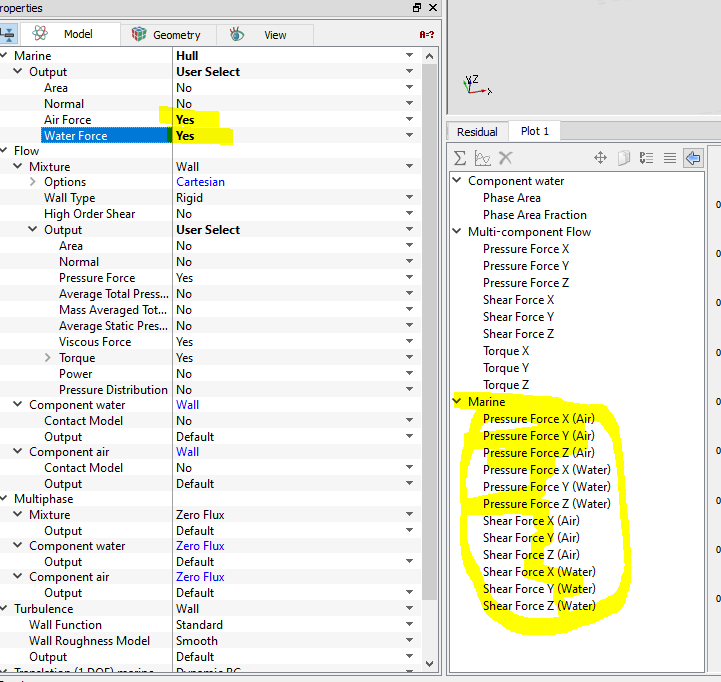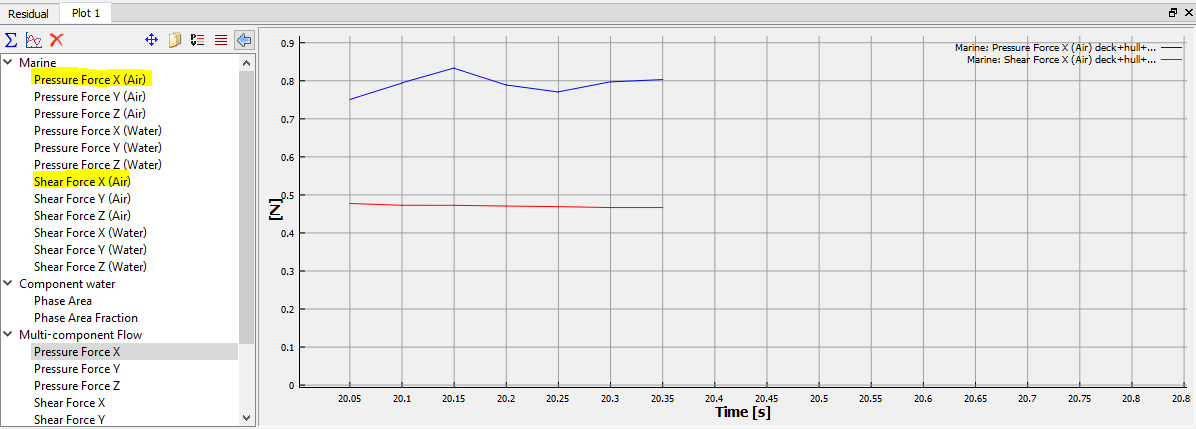As engineers we've been taught that one way to solve a complex problem is to decompose it into a series of simpler problems, and then reconstitute the complex solution by aggregating the simpler solutions. As naval architects we've learned that a practical approach to the problem of predicting the total resistance of a vessel traveling at a constant speed in calm water is to decompose the drag into individual components whose magnitudes can be estimated with rational engineering approaches. For example, it is common to describe the total drag as the sum of frictional resistance (with or without a form factor), residuary resistance (including wavemaking drag) , appendage resistance, and air resistance (not to mention drag due to hull roughness, model-ship correlation allowances, and margins). Each of these components can be estimated though engineering approximations such as regression analyses and empirical/semi-empirical relationships, model experiments, or a combination of these.

It is important to recognize that while this is a very convenient and useful way to approach the problem, this linear decomposition is a simplification of the physics involved. For example, if you have experimental results for a bare hull model of your design and want to add the drag due to the appendages, you might estimate the drag based on model tests of a different design that had the same or similar appendages. However, do you know that the drag increment will be the same if those appendages are added to your design? Will the interaction between the flow around the hull and the appendage be the same for your design as it was on the design from which this data was sourced? We consider this during our analysis and make an engineering judgement as to whether or not we think those effects are significant or can be safely neglected.

When we apply CFD to the resistance problem, the overall problem is not decomposed in the same way. The application of the Navier-Stokes equations means that the fluid flow problem is being solved in a more holistic way. That is not to say that there are not approximations or simplified representations when using CFD. There most certainly are, but many of what some might call the "higher-order" effects of interaction are included in the solution and specifically the effects of fluid viscosity and it's important impacts on the overall problem are being modeled at a much higher fidelity than the linear decomposition approach.

This brings us to the topic of air drag and specifically, in the case of a vessel traveling at constant speed in calm water with no wind, to what is sometimes called still-air drag; air drag that is due solely to the forward motion of the vessel. Traditionally one might make an estimate of the still-air drag by applying a drag coefficient to the transverse frontal area of the vessel above the still waterline. This drag coefficient may be determined experimentally or by comparison to designs with similar above-water shapes and combines the impacts of multiple fluid effects (e.g, friction, flow separation) into one value. Vessels with complex superstructure shapes will typically have a larger drag coefficient than those with a more stream-lined shape. But in any case we end up with a positive increment in drag, that is a value that is resisting the vessel's forward motion.

In a CFD analysis, it would seem that the computation of the still-air drag would be a fairly straightforward process, given that the above-water portion of the model geometry is included in the analysis. Simply integrate the tangential shear and normal pressure forces over all of the cell faces on the vessel that are in contact with the air. For those faces that are at the air/water interface, the cell volume fraction can be used to estimate that portion of the cell face area that is in contact with the air. The longitudinal component of the integrated force yields the air drag. While this all seems straightforward enough, one may end up with results for the air drag that seem non-intuitive or possibly even incorrect. Take the example shown below of a prismatic shape sitting in equilibrium in still water. Because the vessel is stationary there are no viscous shear forces. However, gravitational forces give rise to a pressure distribution that varies vertically with height (relative to a reference height) according to the function rho * g * h. Although, the pressure is not constant, it is easy to see that integration of the pressure forces over the wet and dry faces of the prismatic shape result in no net longitudinal force as expected.Now say that somehow we were able to raise the level of the water forward and simultaneously lower it aft so that it looked like the image below. Here the pressure on the front face would be entirely due to water while that on the aft face would be entirely due to air, and the integration of the pressure forces would be such that the water exerted a net aft-directed force and the air exerted a net forward force. Water being many times denser than air, our vessel would accelerate rearward. Of course while it seems it would take a divine intervention to obtain such a situation at zero speed in calm water, if we consider this prismatic vessel moving with a constant forward velocity the resulting free surface wave pattern results in a similar, though not as extreme, situation.Extending this logic to a more realistic looking vessel shape, albeit foreshortened to accentuate the point, the image below shows a vessel traveling at design speed with the free surface wave intersection shown on the hull. Here the effects of the forward velocity have a significant impact on the pressure distribution in both the air and water fluid phases and additionally the significant effect of viscosity is included so that frictional shear forces abound. However, like the simplified prismatic example above, the existence of the non-horizontal free surface results in what might seem like an imbalance in the integrated longitudinal air and water forces. In particular, the "drying" of the transom adds a significant amount of surface area for which the the net pressure force is likely pointing forward, while the large bow wave reduces the forward-facing area in contact with the air pressure. In certain cases depending on the speed and geometry, this imbalance can skew the reported air component of resistance so that the behavior seems non-intuitive and possibly even in the wrong direction. One can rest assured though that the total drag from the integration of pressure and shear forces over the entire body will give the expected consistent results. The confusion arises out of the interpretation of what is meant by "air drag." In Orca3D Marine CFD the air resistance that is reported from the marine module is obtained by just integrating those cell faces on the vessel that are both in contact with the air and are above the static waterline.Orca3D Marine CFD also allows you to extract the air resistance value that includes all cell faces on the vessel that are in contact with the air (including those that are below the static waterline) as follows:

1. Select all of your vessel geometry boundaries in the Geometric Entities panel as shown below.2. In the Properties panel change the Marine Output to User Select and enable Air and Water forces as shown below.3. Then run a few more time steps and plot the Shear and Pressure forces in the x-direction in air as shown below.Although these two methods of calculating the air resistance will give different results, neither changes the total resistance obtained by summing the longitudinal forces over both the air and water phases, and both might be different from the traditional approach of multiplying the transverse frontal area by a single drag coefficient.